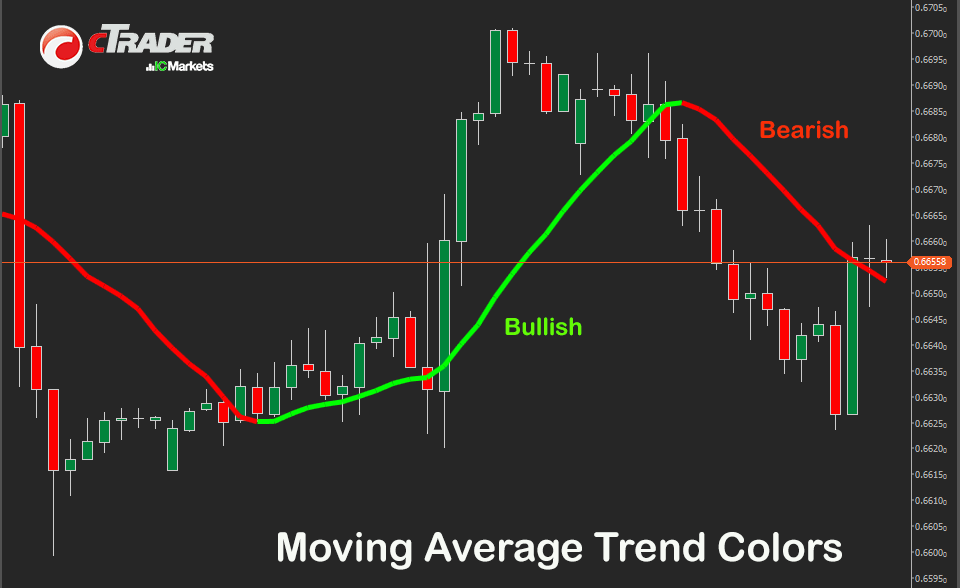# cTrader Moving Average Colors Indicator

This version of the cTrader Moving Average Indicator simply allows you to set the colour of the bullish and bearish line so that you can visually see where the trend is going. You also have the option to choose one of 8 moving average types.
Current version: 2.1.0
Updated: Saturday, 15 July 2023
Author: ClickAlgo Team

Need a broker?  Open a risk-free demo account

£0.00The cTrader moving average is a very popular indicator that is used in technical analysis and it helps smooth out price action by filtering out the “noise” from short-term price changes. Most traders use it as a trend-following or lagging indicator because it is based on past prices. This version of the indicator is different as it shows visually the trend in different colours.

## Moving Average Types

In forex trading, there are several types of moving averages (MA) that traders commonly use to analyze price trends and identify potential entry and exit points. Here are some of the most widely used moving averages in forex:

1. Simple Moving Average (SMA) The simple moving average is the most basic type of moving average. It calculates the average price over a specified period and plots a line on the chart.

2. Exponential Moving Average (EMA) The exponential moving average gives more weight to recent price data compared to older data points. Traders often use EMAs to capture more timely market information and react quickly to changes.

3. Weighted Moving Average (WMA) The weighted moving average assigns different weights to each data point within the selected period. This means that the more recent prices are given greater importance in the calculation.

4. Hull Moving Average (HMA) The Hull moving average is a relatively new type of moving average that aims to reduce lag and provide a smoother curve. It utilizes weighted moving averages and a square root of the period to calculate the values.

5. Time Series Moving Average (TSMA): Time series moving average, also known as time series smoothing or simply moving average, is a statistical technique used to analyze and forecast data points in a time series.

6. Triangular Moving Average (TMA) The triangular moving average is a double-smoothed simple moving average. It applies a simple moving average to a simple moving average, resulting in a triangular-shaped line.

7. Variable Index Dynamic Average (VIDYA) is not a specific moving average calculation but rather a concept introduced by Tushar Chande to adapt the moving average's sensitivity to market volatility. The VIDYA concept involves adjusting the smoothing period of a moving average based on the market's volatility. The goal is to make the moving average more responsive during volatile periods and less sensitive during periods of low volatility.

8. Wilder Moving Average (WMA) is a type of moving average developed by J. Welles Wilder Jr., the creator of popular technical indicators such as the Average True Range (ATR) and the Relative Strength Index (RSI).## How To Install & Remove

First, make sure you have the cTrader trading platform installed and then unzip the file and double-click on it to automatically install it onto the platform.

## Any Questions?

If you have any questions, please first search our coding help forum for the answer, if you cannot find it, post a new question.